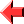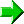﻿ Come Here Tutorial Lesson 3: Variables and Arithmetic#Lesson 3## Using Variables

The only data type is the integer, but there is no defined limit on its size.  A variable name always consists of lowercase letters.  Assignment is done using the `CALL` statement:

``````CALL 30 qwert
CALL qwert*2 yuiop
``````

These two statements assign the value `30` to the variable `qwert`, and then assign twice this value to the variable `yuiop`.

It is illegal to access the value of a variable that hasn't yet been given a value.  Any attempt at doing so will result in a runtime error.

## Arithmetic Operations

Come Here supports the following arithmetic operators:

Symbol Operation Notes
`+` Addition
`-` Subtraction
`*` Multiplication
`//` Integer division Always rounds down.  `5 // 3 = 1` but `(0-5) // 3 = 0-2`
`MOD` Modulo Remainder after division, always has the same sign as the second operand.  `5 MOD 3 = 2` and `(0-5) MOD 3 = 1`
`SGN` Signum `0-1` if operand is negative, `0` if it is `0`, `1` if positive

Note that `-` does not represent unary negation (and neither does `+` represent unary position), since this would introduce a syntactical ambiguity into the language about which little could be done.  To negate a number, subtract it from zero.  Note also that `=` is not a valid Come Here symbol; it is used in the above table, and elsewhere in the tutorial, for purely illustrative purposes.

The operators `*`, `//` and `MOD` all have the same precedence, which is higher than the common precedence of `+` and `-`.  Operators of the same precedence associate from left to right.  So `qwert * 4 + yuiop // 3 - asdfg MOD 2` is equivalent to `((qwert * 4) + (yuiop // 3)) - (asdfg MOD 2)`.  Parentheses can be used to override precedence rules in the same manner as in most languages.

`SGN` has the highest precedence of all operators.  It will prove to be very useful in control flow manipulation, as we will see in the next lesson.